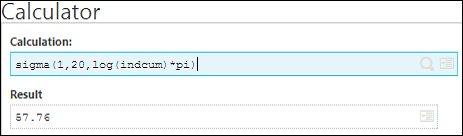# Using Index in Sigma Function in Sage X3

By | May 25, 2017

In Sage X3, the Adonix system variable “indcum” is the total index used by default in the sigma function. The sigma syntax that uses indcum is quicker in execution than the one that uses a variable.

Syntax:

[S]indcum Integer

When you use the sigma function with the following syntax:

sigma(beginning_index,ending_index,expression)

The expression is recalculated for indcum that varies by integers from one(1) to one(1) from the beginning_index value to the ending_index value,inclusive.

Syntax :

sigma(variable=beginning_index ,ending_index ,expression)

Allows you to use any of the user’s variable as a loop variable. This is also used to embed several sigmas recursively.

Example :Index

Indcum is an Integer type variable ; it may therefore only ne used if it varies within the integer range 2^31 and 2^31-1. Moreover sigma expressions may only be nested if the index variable is different from indcum. If the loop variable does not exist, it is created as a Decimal type.

The final value of the index matches the first value outside the given range.